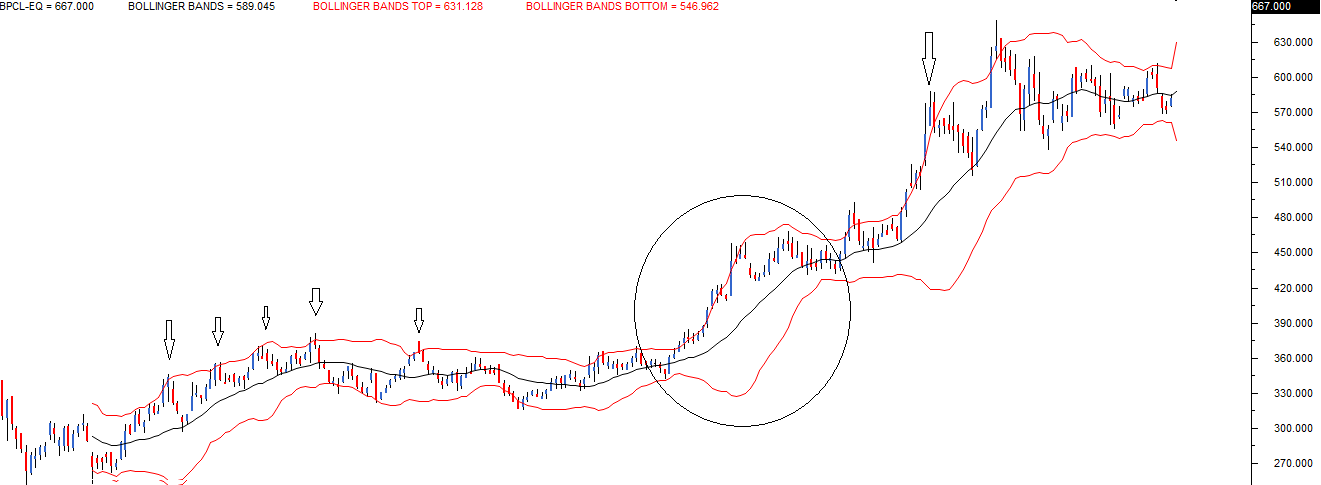## The Bollinger Bands

Introduced by John Bollinger in the 1980s, Bollinger bands (BB) is perhaps one of the most useful indicators used in technical analysis. BB are used to determine overbought and oversold levels, where a trader will try to sell when the price reaches the top of the band and will execute a buy when the price reaches the bottom of the band.
The BB has 3 components:
1. Middle line which is The 20 day simple moving average of the closing prices
2. An upper band – this is the +2 standard deviation of the middle line
3. A lower band – this is the -2 standard deviation of the middle line
The standard deviation (SD) is a statistical concept; which measures the variance of a particular variable from its average. In finance, the standard deviation of the stock price represents the volatility of a stock. For example, if the standard deviation of a stock is 12%, it is as good as saying that the volatility of the stock is 12%.
In BB, the standard deviation is applied on the 20 day SMA. The upper band indicates the +2 SD. By using a +2 SD, we simply multiply the SD by 2, and add it to the average.
For example if the 20 day SMA is 7800, and the SD is 75 (or 0.96%), then the +2 SD would be 7800 + (75*2) = 7950. Likewise, a -2 SD indicates we multiply the SD by 2, and subtract it from the average. 7800 – (2*75) = 7650.
We now have the components of the BB:
1. 20 day SMA = 7800
2. Upper band = 7950
3. Lower band = 7650
Statistically speaking, the current market price should hover around the average price of 7800. However, if the current market price is around 7950, then it is considered expensive with respect to the average, hence one should look at shorting opportunities with an expectation that the price will scale back to its average price.
Therefore the trade would be to sell at 7950, with a target of 7800.
Likewise if the current market price is around 7650, it is considered cheap with respect to the average prices, and hence one should look at buying opportunities with and expectation that the prices will scale back to its average price.
Therefore the trade would be to buy at 7650, with a target of 7800.
The upper and lower bands act as a trigger to initiate a trade.
The following is the chart of BPCL Limited,The central black line is the 20 day SMA. The two red lines placed above and below the black like are the +2 SD, and -2SD. The idea is to short the stock when the price touches the upper band with an expectation that it will revert to average. Likewise one can go long when the price touches the lower band with an expectation it will revert to the average.
I have highlighted using a down arrow all the sell signals BB generated, while most of the signals worked quite well, there was a phase when the price stuck to the upper band. In fact the price continued to drift higher, and therefore even the upper band expanded. This is called an envelope expansion.
The BB’s upper and lower band together forms an envelope. The envelope expands, whenever the price drifts in a particular direction indicating a strong momentum. The BB signal fails when there is an envelope expansion. This leads us to an important conclusion; BB works well in sideways markets, and fails in a trending market.
Personally whenever, I use BB I expect the trade to start working in my favor almost immediately. If it does not, I start validating the possibility of an envelope expansion.